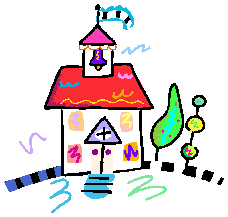# LEVITTOWN PUBLIC SCHOOLS

 TermsFriday, Oct. 22, 2021 Today Is: Day 2ABBEYDIVISIONEAST BROADWAYGARDINERSLEE ROADMACARTHURNORTHSIDESALKSUMMITWISDOM

## PARENTS

### Best Math Resources For Fourth - Fifth GradeThis list was the product of an Independent Study Group through the Levittown Teacher's Center. The sites were chosen and organized by a group of Gardiners Avenue Teachers and Teaching Assistants.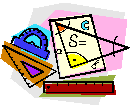#### Number Sense and Operations: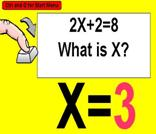#### Algebra

Variables, equations and expressions
Patterns, relations and functions

#### Geometry

Shapes
Area, perimeter
Line, line relationships and angles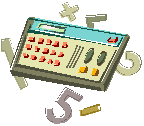#### Measurements

Linear, capacity, mass
Time
Money

#### Logic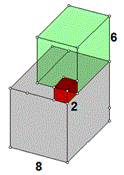#### Math Online Resources:

Math Dictionary for Kids - Animated, interactive dictionary that illustrates and explains over 500 mathematical principals and gives examples for you to complete.

That Quiz
Resource for math practice and testing

Areas include:

Integers: Arithmetic, Inequality, Exponents, Algebra and Calculus

Fractions: Identify, Arithmetic, Inequality, Reduce and Probability

Concepts: Time, Money, Measure, Place value and Graphs

Geometry: Triangles, Shapes, Geometry, Points, Angles

Rainforest Math - Interactive math activities
www.rainforestmaths.com

Go to Main Menu, choose your grade and select the math concept you want to work on.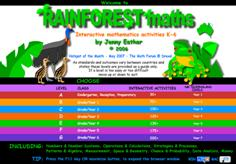Categories include:
Numbers: Place value, Ordering, Rounding, Operations and Calculation & Fractions
Algebra: Patterns, Functions, Equivalence, and Equations
Measurement: Length, Area, Volume, Capacity, Mass and Time
Space: Shapes and Position
Chance : Probability
Data: Graphs
Money